# Link Connection Points on Nodes

There is flexibility in controlling exactly how and where a link connects to a node. In the previous examples the link has always ended at the edge of the node. But you can specify the Spot on a node at which a link terminates.

## Non-rectangular Nodes

When a Node does not have a rectangular shape, by default links will end where the line toward the center of the node intersects with the edge of the node.

Here is a demonstration of that -- drag one of the nodes around and watch how the link always connects to the nearest intersection or to the center of the node. This example includes arrowheads at both ends of the link, to make it clear that the link route really ends right at the edge of the node.

``````
diagram.NodeTemplate =
new Node("Auto") {
Width = 90, Height = 90,
}
.Bind("Location", "Loc", Point.Parse)
new Shape("FivePointedStar") { Fill = "lightgray" },
new TextBlock().Bind("Text", "Key")
);

new Shape(),
new Shape { FromArrow = "Chevron" },  // the "from" end arrowhead
new Shape { ToArrow = "StretchedDiamond", Fill = "red" }  // the "to" end arrowhead
);

diagram.Model =
new MyModel {
NodeDataSource = new List<NodeData> {
new NodeData { Key = "Alpha", Loc = "0 0" },
new NodeData { Key = "Beta", Loc = "100 50" }
},
new LinkData { From = "Alpha", To = "Beta" }
}
};
``````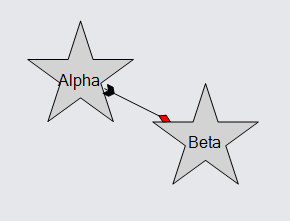## ToSpot and FromSpot

You can easily require links to end at a particular point within the bounds of the node, rather than at the nearest edge intersection. Set the GraphObject.ToSpot to a Spot value other than Spot.None to cause links coming into the node to end at that spot within the node, with a direction that is appropriate for the side that the spot is at. Similarly, set the GraphObject.FromSpot for the ends of links coming out of the node.

The following examples all display the same graph but use different templates to demonstrate how links can connect to nodes. They all call this common function to define some nodes and links.

``````
private void MakeGraph() {
diagram.Layout =
new LayeredDigraphLayout {
ColumnSpacing = 5, SetsPortSpots = false
};

diagram.Model =
new MyModel {
NodeDataSource = new List<NodeData> {
new NodeData { Key = "Alpha" }, new NodeData { Key = "Beta" },
new NodeData { Key = "Gamma" }, new NodeData { Key = "Delta" },
new NodeData { Key = "Epsilon" }, new NodeData { Key = "Zeta" },
new NodeData { Key = "Eta" }, new NodeData { Key = "Theta" }
},
new LinkData { From = "Beta", To = "Alpha" },
new LinkData { From = "Gamma", To = "Alpha" },
new LinkData { From = "Delta", To = "Alpha" },
new LinkData { From = "Alpha", To = "Epsilon" },
new LinkData { From = "Alpha", To = "Zeta" },
new LinkData { From = "Alpha", To = "Eta" },
new LinkData { From = "Alpha", To = "Theta" }
}
};
}
``````

Let us specify that links coming into a node connect at the middle of the left side, and that links going out of a node connect at the middle of the right side. Such a convention is appropriate for diagrams that have a general sense of direction to them, such as the following one which goes from left to right.

``````
diagram.NodeTemplate =
new Node("Auto") {
FromSpot = Spot.Right,  // coming out from middle-right
ToSpot = Spot.Left  // going into at middle-left
}
new Shape { Fill = "lightgray" },
new TextBlock { Margin = 5 }
.Bind("Text", "Key")
);

new Shape(),
new Shape { ToArrow = "Standard" }
);

MakeGraph();
``````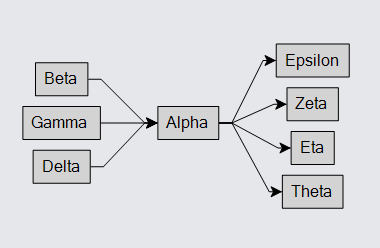You can also specify that the links go into a node not at a single spot but spread out along one side. Instead of Spot.Right use Spot.RightSide, and similarly for the left side.

``````
diagram.NodeTemplate =
new Node("Auto") {
FromSpot = Spot.RightSide,  // coming out from right side
ToSpot = Spot.LeftSide  // going into left side
}
new Shape { Fill = "lightgray" },
new TextBlock { Margin = 5 }
.Bind("Text", "Key")
);

new Shape(),
new Shape { ToArrow = "Standard" }
);

MakeGraph();
``````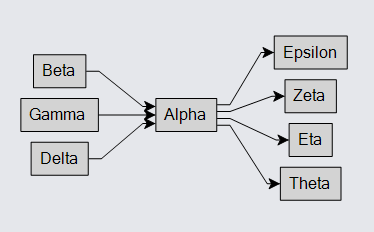Of course this only looks good when the nodes are basically rectangular.

You can use a different kind of Link.Routing:

``````
diagram.NodeTemplate =
new Node("Auto") {
FromSpot = Spot.RightSide,  // coming out from right side
ToSpot = Spot.LeftSide  // going into left side
}
new Shape { Fill = "lightgray" },
new TextBlock { Margin = 5 }
.Bind("Text", "Key")
);

Routing = LinkRouting.Orthogonal,  // Orthogonal routing
Corner = 5  // with rounded corners
}
new Shape(),
new Shape { ToArrow = "Standard" }
);

MakeGraph();
``````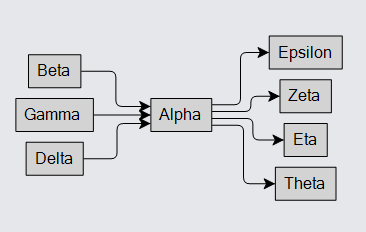Or you can use a different kind of Link.Curve:

``````
diagram.NodeTemplate =
new Node("Auto") {
FromSpot = Spot.RightSide,  // coming out from right side
ToSpot = Spot.LeftSide  // going into left side
}
new Shape { Fill = "lightgray" },
new TextBlock { Margin = 5 }
.Bind("Text", "Key")
);

new Shape(),
new Shape { ToArrow = "Standard" }
);

MakeGraph();
``````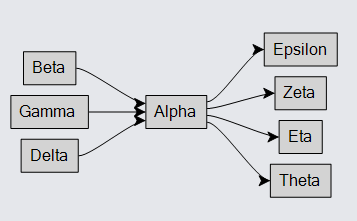But you need to be careful to specify sensible spots for how the graph is arranged.

``````
diagram.NodeTemplate =
new Node("Auto") {
FromSpot = Spot.TopSide,  /// coming out from top side -- BAD!
ToSpot = Spot.RightSide  // going into right side -- BAD!
}
new Shape { Fill = "lightgray" },
new TextBlock { Margin = 5 }
.Bind("Text", "Key")
);

new Shape(),
new Shape { ToArrow = "Standard" }
);

MakeGraph();

new Part { Location = new Point(300, 50) }  // this is just a comment
new TextBlock("Bad Spots") { Font = new Font("Segoe UI", 16, FontWeight.Bold), Stroke = "red" }
)
);
``````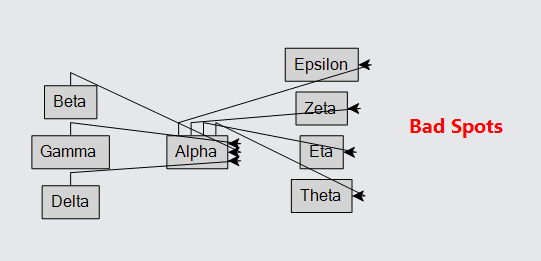### Undirected Spots

When no spot is specified for the GraphObject.FromSpot or GraphObject.ToSpot, the route computation will compute the furthest point on the route of the link from the center of the port to the other port that is an intersection of an edge of the port. This was demonstrated above in Non-rectangular Nodes and is again demonstrated here.

``````
diagram.NodeTemplate =
new Node("Vertical")
.Bind("Location", "Loc", Point.Parse)
new Shape("YinYang") { Fill = "white", PortId = "" }
.Bind("Fill", "Color"),
new TextBlock { Margin = 8 }
.Bind("Text", "Key")
);

diagram.Model =
new MyModel {
NodeDataSource = new List<NodeData> {
new NodeData { Key = "Alpha", Color = "lightblue", Loc = "0 50" },
new NodeData { Key = "Beta", Color = "orange", Loc = "150 0" },
new NodeData { Key = "Gamma", Color = "lightgreen", Loc = "300 50" }
},
new LinkData { From = "Alpha", To = "Beta" },
new LinkData { From = "Beta", To = "Gamma" },
}
};
``````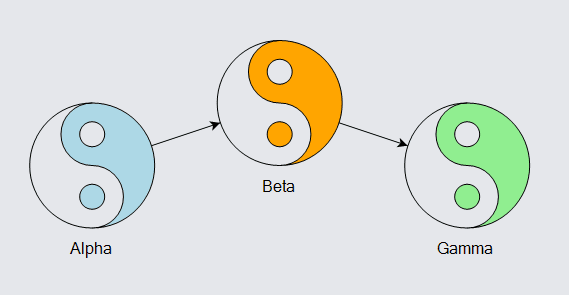However it is possible to specify a focus point that is different from the center of the port. Use a Spot value that has Spot.X and Spot.Y equal to 0.5 but with Spot.OffsetX and Spot.OffsetY values that specify where you want links to focus towards, relative to the center of the port.

``````
diagram.NodeTemplate =
new Node("Vertical")
.Bind("Location", "Loc", Point.Parse)
new Shape("YinYang") {
Fill = "white", PortId = "",
FromSpot = new Spot(0.5, 0.5, 0, -25), ToSpot = new Spot(0.5, 0.5, 0, 25)
}
.Bind("Fill", "Color"),
new TextBlock { Margin = 8 }
.Bind("Text", "Key")
);

diagram.Model =
new MyModel {
NodeDataSource = new List<NodeData> {
new NodeData { Key = "Alpha", Color = "lightblue", Loc = "0 50" },
new NodeData { Key = "Beta", Color = "orange", Loc = "150 0" },
new NodeData { Key = "Gamma", Color = "lightgreen", Loc = "300 50" }
},
new LinkData { From = "Alpha", To = "Beta" },
new LinkData { From = "Beta", To = "Gamma" },
}
};
``````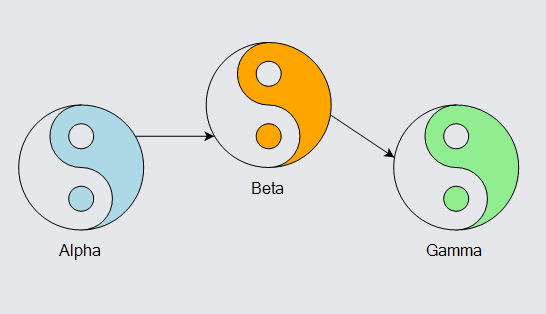In this example, links always appear to be coming from the hole near the top of the "YinYang" figure towards the dot near the bottom of the figure. Note that the Spot.X and Spot.Y values are both 0.5, with fixed offsets from the center of the port.

It is also possible to have links go directly to particular spots within a port. Use regular Spot values, but set the Link's end segment length to zero, Link.FromEndSegmentLength or Link.ToEndSegmentLength.

``````
diagram.NodeTemplate =
new Node("Vertical")
.Bind("Location", "Loc", Point.Parse)
new Shape("YinYang") {
Fill = "white", PortId = "",
FromSpot = new Spot(0.5, 0.25), ToSpot = new Spot(0.5, 0.75),
FromEndSegmentLength = 0, ToEndSegmentLength = 0
}
.Bind("Fill", "Color"),
new TextBlock { Margin = 8 }
.Bind("Text", "Key")
);

diagram.Model =
new MyModel {
NodeDataSource = new List<NodeData> {
new NodeData { Key = "Alpha", Color = "lightblue", Loc = "0 50" },
new NodeData { Key = "Beta", Color = "orange", Loc = "150 0" },
new NodeData { Key = "Gamma", Color = "lightgreen", Loc = "300 50" }
},
new LinkData { From = "Alpha", To = "Beta" },
new LinkData { From = "Beta", To = "Gamma" },
}
};
``````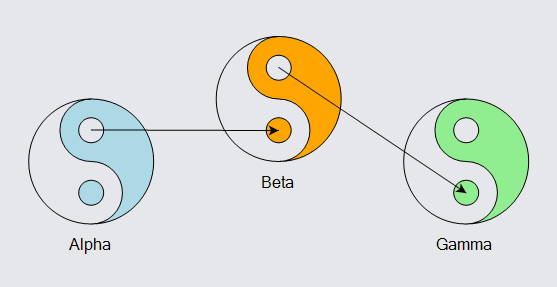Again, links always appear to be coming from the hole near the top of the "YinYang" figure towards the dot near the bottom of the figure, but now they go all the way rather than stop at the edge. Note that the Spot.X and Spot.Y values are not both 0.5, and that the Link end segment lengths are zero.

Setting the GraphObject.FromSpot and GraphObject.ToSpot properties specifies the default link connection point for all links connected to the node. What if you want some links to go to the middle-top spot but some other links to go to the middle-left spot of the same node? You can achieve this by setting the Link.FromSpot and Link.ToSpot properties, which take precedence over the correspondingly named properties of what the link connects with.

``````
diagram.NodeTemplate =
new Node("Auto")
new Shape { Fill = "lightgray" },
new TextBlock { Margin = 5 }
.Bind("Text", "Key")
);

.Bind("FromSpot", Spot.Parse)
.Bind("ToSpot", Spot.Parse)
new Shape(),
new Shape { ToArrow = "Standard" }
);

diagram.Model =
new MyModel {
NodeDataSource = new List<NodeData> {
new NodeData { Key = "Alpha" }, new NodeData { Key = "Beta" },
new NodeData { Key = "Gamma" }, new NodeData { Key = "Delta" }
},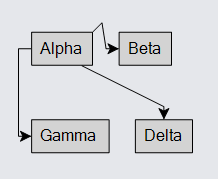Some of the predefined Layouts automatically set Link.FromSpot and Link.ToSpot when the nature of the layout implies a natural direction. So, for example, a TreeLayout with a TreeLayout.Angle `== 90` will set each Link's FromSpot to be Spot.Bottom and each Link's ToSpot to be Spot.Top.